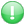Get premium membership and access revision papers, questions with answers as well as video lessons.

# Bsm 211: Microeconomics Question Paper

Bsm 211: Microeconomics

Course:Bachelor Of Science In Strategic Management

Institution: Moi University question papers

Exam Year:2017

DEPARTMENT OF DEVELOPMENT STUDIES
2ndYEAR 2nd SEMESTER EXAMINATIONS
BSc. IN STRATEGIC MANAGEMENT
BSM 211: MICROECONOMICS

Instructions to Candidates:
?Section A is COMPULSORY
?Answer ANY THREE Questions in section B
?TIME:3 HOURS

SECTION A: COMPULSORY

Q1. a)With the aid of diagrams,explain
the law of variable proportions. Examine the relationship between total product (TP),average product (AV)and marginal product (MP). (15 mks)
b) Briefly discuss the factors which lead to increasing and decreasing returns to scale in the production process. (10mks)

Q2. a) with the aid of a diagram, explain the concept of a firm's least cost factor combination. (9mks)
b) Explain the following economic concepts:
i)Marginal rate of technical substitution. (3mks)
ii)Isocost lines. (3mks)
Q3. a) With the help of a well labeled diagram, explain the relationship between the average fixed cost, average variable cost, average total cost curves and marginal cost curves. (10mks)
b) Explain why the marginal cost curves and average cost curves are U-shaped. (5mks)
Q4. Using appropriate diagrams, explain the properties of an isoquant. (15mks)
Q5. a) Distinguish between economies and diseconomies of scale and examine the types of internal and external economies of scale. (9mks)
b) Outline the significance of mobility of factors of production in an economy. (6mks)
Q6. Discuss the factors responsible for wage differentials between occupations and within the same occupations in the labour market and suggest measures to mitigate the situation. (15mks)

XXXENDXXX